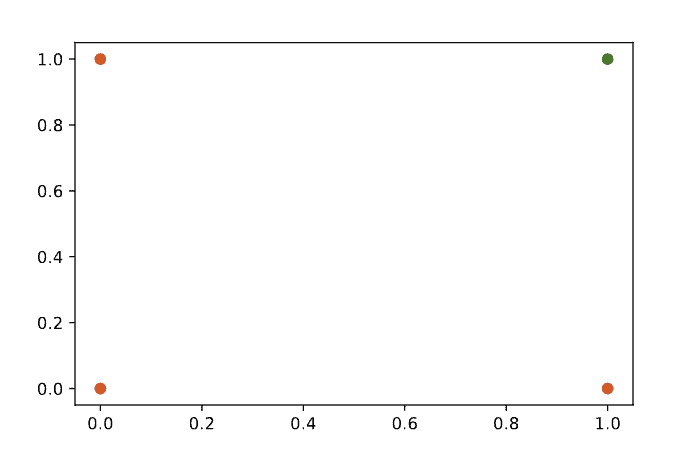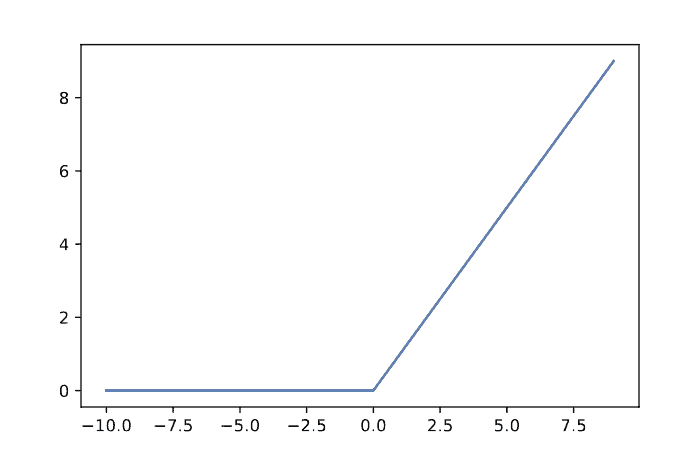## 1. Introduction

Activation functions, also called non-linearities, are an important part of neural network structure and design, but what are they? We explore the need for activation functions in neural networks before introducing some popular variants. We explain the utility and history of the most popular activation functions. Finally, we provide tips on deciding which is best for your application.

## 2. What Are Activation Functions?

Before we discuss activation functions, we first recall the structure of a standard feed-forward neural network.

We start with a single layer. This layer is a standard linear transformation of the input,, given weightsand bias.

This is a standard linear regression model at this point. We can stack multiple layers to create a neural network, and between those layers, we place activation functions.

A simple neural network contains two such transformations. One layer transforms the input into the hidden layer representation, and one layer transforms the hidden layer into the output. A non-linear activation follows the first transformation. This formulation is very powerful and can approximate any function to an arbitrary level of precision.

How is this simple network so powerful? What is a non-linear activation function, and why is it so important? Let us explore this with an example.

### 2.1. Classification: an Example

Think about a classification problem that aims to predict a single boolean value. For example, is this the result of applying this function, True or False? To be more concrete, we can think about the AND function, a linearly separable function. Below, a scatter plot of the result of the AND function for each of its inputs is shown. We can see how we can draw a straight line to separate the True (green) and False (red) outputs:If we consider just a single-layer network, we have the base model of the logistic regression algorithm. Our model can predict a number for each input data point, and we set a threshold. If the prediction is above the threshold, predict True; otherwise, predict False. Suppose we wrote “if” as a function, it would be a step function. A type of discontinuous activation function. Since we want to talk about activation functions for neural networks and because we want to train our network using the backpropagation algorithm, we need to introduce a continuous version of the binary step function we just discussed. We do this because backpropagation requires differentiable functions.

The Sigmoid function is our continuous step function. It squashes all values into the range [0,1] with a very steep interval such that most values are close to either 0 or 1.

This is what an activation function is. An activation function is a non-linear transformation of its input data. As we have just seen, this can be useful in the output layer, but it is also helpful in the body of a deeper network.

## 3. Why Use an Activation Function?

In the previous section, we discussed the use of an activation function to produce an almost binary classification signal. However, non-linearities are also essential as part of deeper neural networks. This section provides further context, highlighting how most problems are non-linear and how neural networks are intrinsically linear without the non-linear activation functions.

### 3.1. Most Problems Are Non-linear

The most basic reason is that many prediction problems do not have linear outputs. Many curves are defined by some form of polynomial or more complex function. Even tasks that sound easy can require non-linear decision boundaries. We consider the boundary required to classify the output for the logical XOR function. It can’t be done with a single straight line!

It can be shown that neural networks are universal function approximators; like most theorems of this nature, it is best not to read too much into this. Further, without a non-linear activation, a neural network is a composition of linear transformations of the data and, therefore, linear. By interleaving the neural network layer with activation functions, we can produce highly non-linear and complex decision boundaries.

### 3.2. Going Deeper

Activation functions are also integral when it comes to building deeper networks. The learning dynamics of deep neural networks are complex, and many aspects of training require tuning.

Training neural networks rely on the gradient signal flowing through the network. A problem with the traditional Sigmoid activation is that it saturates close to its extremes and produces very small gradients. In larger networks, this can cause the signal to be slow to propagate. To alleviate this and other issues, many types of activation functions are proposed in the literature.

## 4. Common Activation Functions

Several different activation functions have been used in neural networks as the field has developed. Some functions, developed to help solve specific issues, are now more common than their previously popular predecessors.

Two early favorites were the S-shaped curves of the Sigmoid and TanH functions.

Sigmoid and TanH activations are also used in recurrent architectures where they are used in defining the working of LSTM and GRU cells. Although Sigmoid and TanH are popular, they tend to saturate near their maximum and minimum values. This makes the activation functions sensitive to their inputs only for a very small range of values. In deeper neural networks, this can lead to the vanishing gradient problem. The next activation function we discuss can alleviate that issue.

### 4.1. ReLU

Rectified Linear Unit is now a popular and standard activation function. Despite its linear appearance, the ReLU is highly utilized in neural networks and can help to avoid the vanishing gradient problem that occurs for very large or very small values passed to either TanH or Sigmoid activations. A problem with ReLU is the potential for dead neurons. Leaky-ReLU is also popular to alleviate this issue, as it leaves small non-zero signal flow through the activation.

We define the ReLU activation function as:.

We can see it in the image below:### 4.2. Other Activations

There are many other activation functions to choose from, often with more specific use cases. It is also possible to define your own activation function.

Certain cases may require a specific output shape, as in our classification example and the use of sigmoid to produce an (almost) binary output. Other use cases might invoke a periodic activation function if that is the nature of the output signal. Such an approach is a way to bias or add a prior to your model.

## 5. Conclusion

In this article, we have seen how activation functions are an essential part of neural network architecture. The development of neural network architectures has prompted the development of new activation functions more suited to learning dynamics.

Even a superficial, single hidden layer neural network paired with a single non-linearity is extremely powerful. While the choice of non-linearity may seem daunting, it is best to start with the current standard of ReLU and to experiment from there if you fail to achieve the desired result.